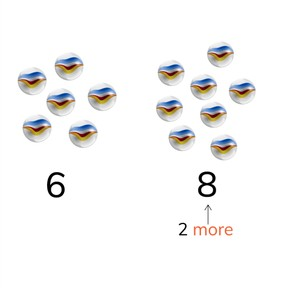Determining difference to 20

# Determining difference to 20

No account needed.8,000 schools use Gynzy92,000 teachers use Gynzy1,600,000 students use Gynzy

## General

Students learn to determine whether numbers to 20 are greater or less than each other. They do so by creating addition and subtraction problems.

1.OA.C

## Introduction

The interactive whiteboard shows two images. Ask students to determine what the subtraction problem is that is being shown, and ask them to find the difference.

## Instruction

Explain to students that when you want to determine difference to 20 you look to see if a number is larger or smaller than the other. Demonstrate this by counting the fish in the fishbowl. Ask students to determine how many more fish are in the one fishbowl as compared to the other fishbowl. Then explain that you can determine how large a gap is between two numbers by using the number line. Show students the two number lines and two ways in which to count to see what the difference between the two numbers is. Next explain that you can also determine the difference between the two numbers by creating a subtraction problem. You can use the numbers 12 and 8 to make the subtraction problem 12 - 8 to find the difference. If both of the numbers are greater than 10, you can use simplification to solve the problem. Discuss with students that you can also create an addition problem to solve the difference. Finally show students a story problem and explain the steps they need to solve the difference between the two numbers. Ask students to solve a story problem in groups/pairs/individually and then discuss how they got to their answers.

Check that students understand determining difference to 20 by asking the following questions:
- How can you determine the difference between two given numbers? (make an addition or subtraction problem)
- Which subtraction problem do you create to determine the difference between the numbers 9 and 14?
- What must you pay attention to when working with a story problem?

## Quiz

Students first practice determining the difference between two given numbers with visual support in the form of blocks. Then they get two numbers and are asked to determine the difference without the visual support. They then get a story problem in which they must determine what the original total was after a given number has been taken away.

## Closing

Repeat the learning goal and why it is important for students to learn how to determine difference. The interactive whiteboard now shows two kids talking about how many goals they made. Students must determine the difference. They must do the same for the two kids talking about how many points they scored in their card game. Ask students to explain how they determined the difference between the two given numbers. You can do so by having them discuss this in pairs and then sharing with the class.

## Teaching tips

Students who have difficulty with this learning goal can be supported by the use of blocks or other manipulatives that they can use to represent the numbers given. Students can make two piles to represent the two given numbers and then determine how they want to find the difference.

## Instruction materials

optional- blocks or other manipulatives

### The online teaching platform for interactive whiteboards and displays in schools

• Save time building lessons

• Manage the classroom more efficiently

• Increase student engagement